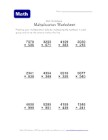This set of printable multiplication worksheets contains lots of problems that involve multiplying multi-digit numbers. This is our hardest set of multiplication worksheets for kids so far, so this isn't for the kids just starting out with learning to multiply (check out our other versions of multiplication worksheets for beginners). These multiplication worksheets are printable and great for any child that already knows how to multiply single and two digit numbers and is looking for something a little more advanced.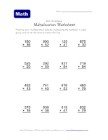## Multiple Digit Multiplication Worksheet 1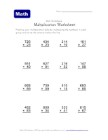## Multiple Digit Multiplication Worksheet 2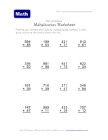## Multiple Digit Multiplication Worksheet 3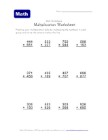## Multiple Digit Multiplication Worksheet 4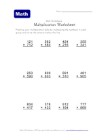## Multiple Digit Multiplication Worksheet 5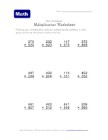## Multiple Digit Multiplication Worksheet 6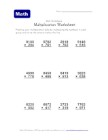## Multiple Digit Multiplication Worksheet 7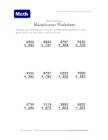## Multiple Digit Multiplication Worksheet 8